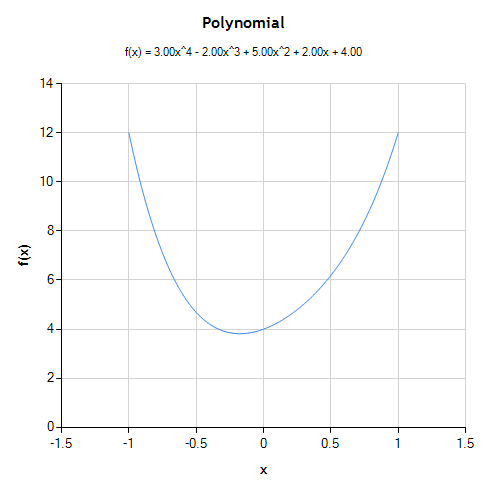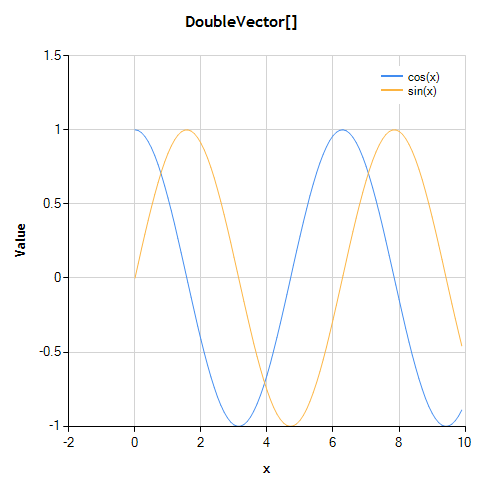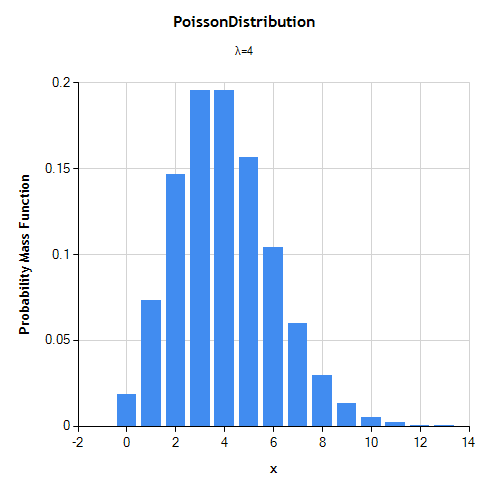# .NET Math with Microsoft Chart Controls, Revisited

### Easy Math Charting

In an earlier post, we described how NMath types can be plotted using the Microsoft Chart Controls for .NET, but programmatically constructing Chart objects with data series bound to NMath objects. The latest release of the NMath libraries makes this process much simpler by providing adapter classes which easily construct basic math charts for you from NMath types:

• NMath 5.1 includes assembly NMathChartMicrosoft.dll containing class NMathChart, which provides convenience methods for plotting NMath types using the Microsoft Chart Controls
• NMath Stats 3.4 includes assembly NMathStatsChartMicrosoft.dll containing NMathStatsChart which provides convenience methods for plotting NMath Stats types using the Microsoft Chart Controls

The generated charts can then be customized to meet your needs.

### Using the Math Chart Adapters

The Microsoft Chart Controls for .NET are available as a separate download for .NET 3.5. Beginning in .NET 4.0, the Chart controls are part of the .NET Framework. To use the Chart controls, add a reference to `System.Windows.Forms.DataVisualization`.
To use the adapters, add a reference to NMathChartMicrosoft.dll and/or NMathStatsChartMicrosoft.dll, and `using` statement:

`using CenterSpace.NMath.Charting.Microsoft;`

A previous blog article provides a simple `app.config` solution for deploying your chart dependent applications to target both .NET 3.5 or .NET 4.0. Without this `app.config` modification, your application will only target .NET 3.5.

### The Math Chart Adapter API

Both NMathChart and NMathStatsChart classes share a common API. Overloads of the `ToChart()` function are provided for our common math and stats types. `ToChart()` returns an instance of `System.Windows.Forms.DataVisualization.Charting.Chart`, which can be customized as desired. For example

```Polynomial poly = new Polynomial( new DoubleVector( 4, 2, 5, -2, 3 ) );
Chart chart = NMathChart.ToChart( poly, -1, 1 );

For prototyping and debugging console applications, the `Show()` function shows a given chart in a default form.

`NMathChart.Show( chart );`

Note that when the window is closed, the chart is disposed.

If you do not need to customize the chart, overloads of `Show()` are also provided for common NMath types.

`NMathChart.Show( poly );`

This is equivalent to calling:

`NMathChart.Show( NMathChart.ToChart( poly ) );`

The `Save()` function saves a chart to a file or stream.

`NMathChart.Save( chart, "chart.png", ChartImageFormat.Png );`

If you are developing a Windows Forms application using the Designer, add a Microsoft Chart control to your form, then update it with an NMath object using the appropriate `Update()` function after initialization.

```public Form1()
{
InitializeComponent();

Polynomial poly =
new Polynomial( new DoubleVector( 4, 2, 5, -2, 3 ) );
NMathChart.Update( ref this.chart1, poly, -1, 1 );
}```

This has the following effect on your existing Chart object:

• a new, default ChartArea is added if one does not exist, otherwise chart.ChartAreas is used
• axis titles, and DefaultAxisTitleFont and DefaultMajorGridLineColor, only have an effect if a new ChartArea is added
• titles are added only if the given Chart does not already contain any titles
• chart.Series is replaced, or added if necessary

### Charting Examples

Here are a few examples of usage in C# (Of course, as with all NMath functionality, these examples will also work, given the necessary syntactic changes, from VB.NET or F#, or any other .NET language.) :

```double xmin = -1;
double xmax = 1;
int numInterpolatedValues = 100;
Polynomial poly = new Polynomial( new DoubleVector( 4, 2, 5, -2, 3 ) );
NMathChart.Show( poly, xmin, xmax, numInterpolatedValues );``````DoubleVector x = new DoubleVector( 100, 0, 0.1 );
DoubleVector cos = x.Apply( NMathFunctions.CosFunction );
DoubleVector sin = x.Apply( NMathFunctions.SinFunction );

DoubleVector[] data = new DoubleVector[] { cos, sin };
NMathChart.Unit unit = new NMathChart.Unit( 0, 0.1, "x" );
Chart chart = NMathChart.ToChart( data, unit );

chart.Series.Name = "cos(x)";
chart.Series.Name = "sin(x)";
NMathChart.Show( chart );``````double lambda = 4.0;
PoissonDistribution poisson = new PoissonDistribution( lambda );

NMathStatsChart.Show( poisson,
NMathStatsChart.DistributionFunction.PDF );``````DoubleMatrix predictors =
new DoubleMatrix( 100, 3, new RandGenUniform() ); ;
DoubleVector observations = 2 * predictors.Col(0) +
-0.75 * predictors.Col(1) + 1.25 * predictors.Col(2) + 0.5;
``